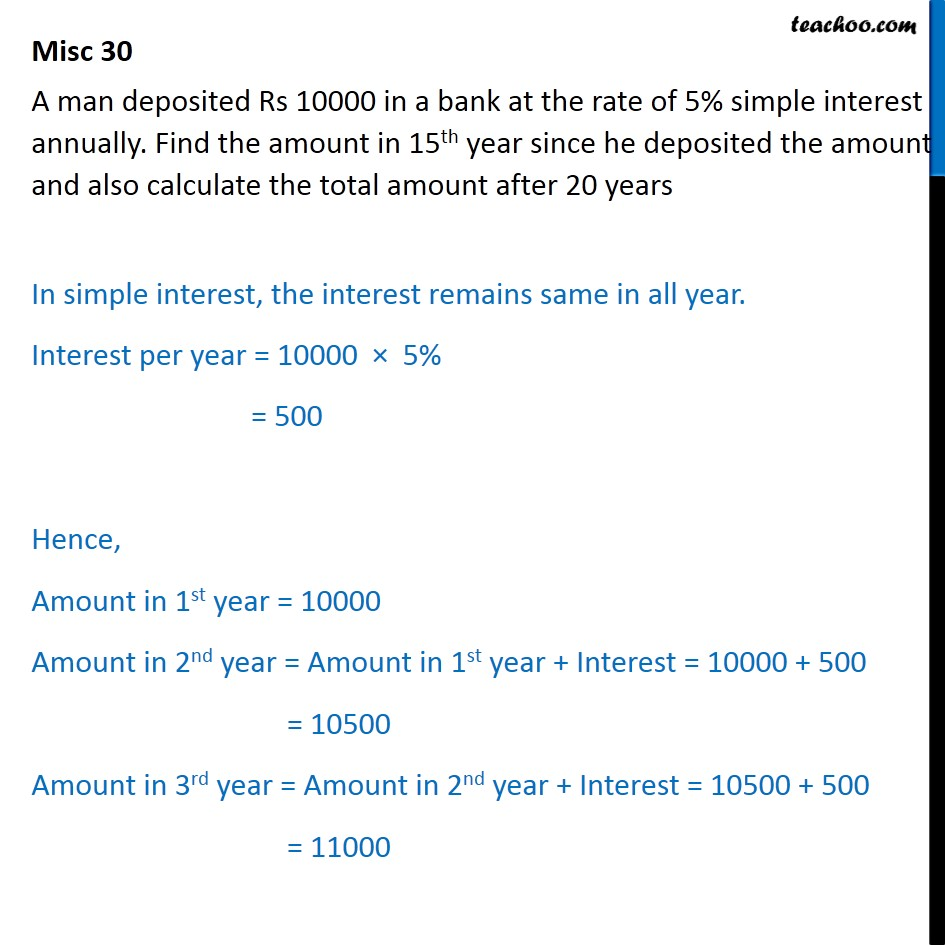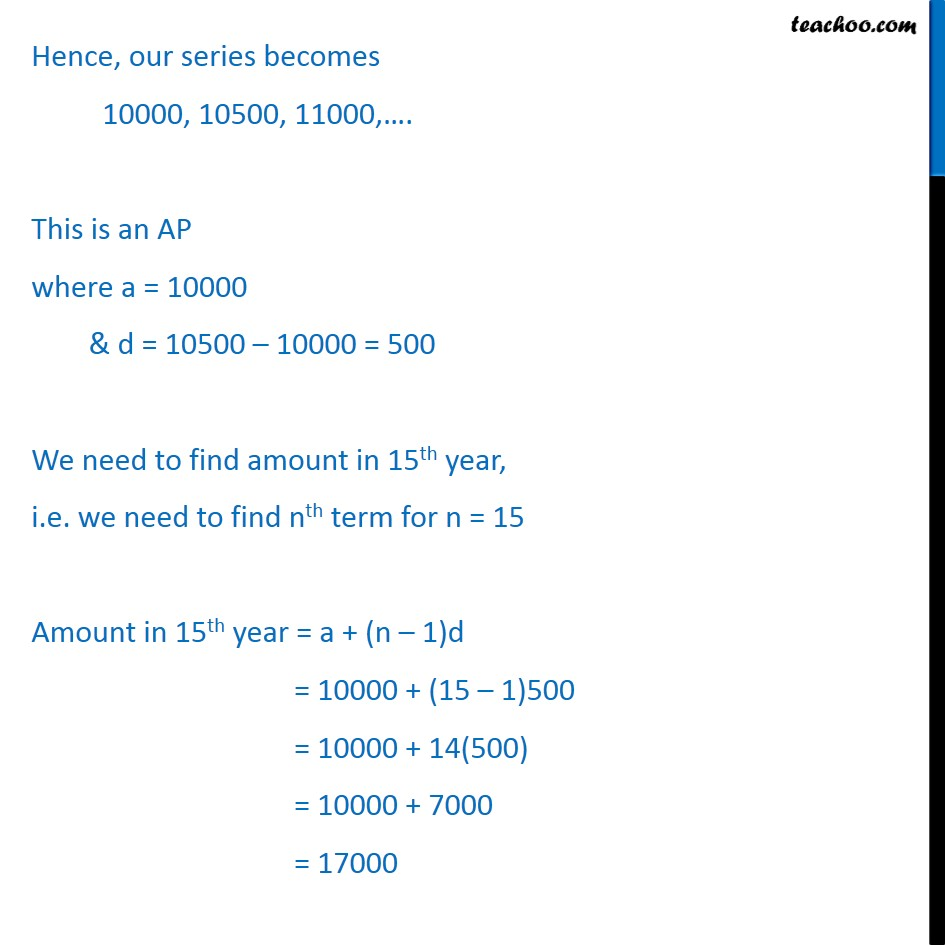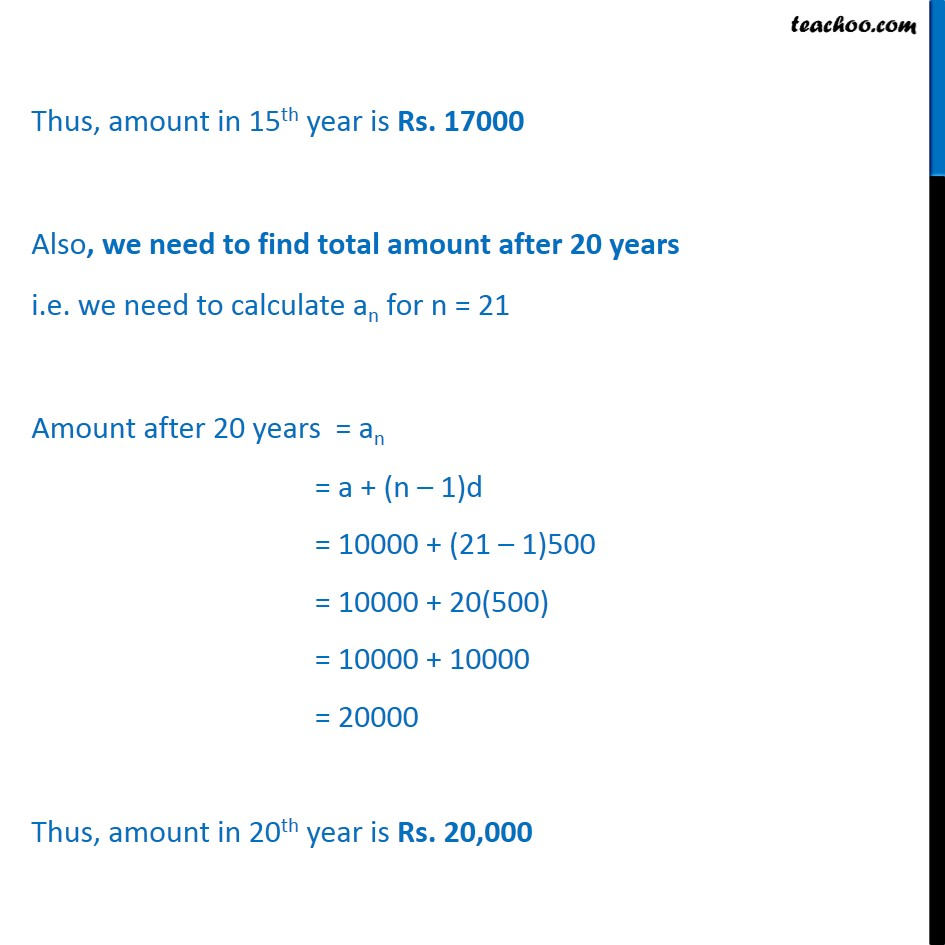Miscellaneous

Chapter 9 Class 11 Sequences and Series (Term 1)
Serial order wise### Transcript

Misc 30 A man deposited Rs 10000 in a bank at the rate of 5% simple interest annually. Find the amount in 15th year since he deposited the amount and also calculate the total amount after 20 years In simple interest, the interest remains same in all year. Interest per year = 10000 5% = 500 Hence, Amount in 1st year = 10000 Amount in 2nd year = Amount in 1st year + Interest = 10000 + 500 = 10500 Amount in 3rd year = Amount in 2nd year + Interest = 10500 + 500 = 11000 Hence, our series becomes 10000, 10500, 11000, . This is an AP where a = 10000 & d = 10500 10000 = 500 We need to find amount in 15th year, i.e. we need to find nth term for n = 15 Amount in 15th year = a + (n 1)d = 10000 + (15 1)500 = 10000 + 14(500) = 10000 + 7000 = 17000 Thus, amount in 15th year is Rs. 17000 Also, we need to find total amount after 20 years i.e. we need to calculate an for n = 21 Amount after 20 years = an = a + (n 1)d = 10000 + (21 1)500 = 10000 + 20(500) = 10000 + 10000 = 20000 Thus, amount in 20th year is Rs. 20,000